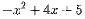# Feb 24th: Interpreting Quadratic function

## Your Turn:

1. Input the quadratic function g(x) = 2. Specify special points such as vertex, roots and y-intercept. 3. Draw the line of symmetry. 4. Plot 2 pairs of points that reflect across the line of symmetry. 5. Generate the table of values for g(x).

## Show your graph here:

1. Which of the following describes the vertex of g(x)?

Check all that apply

2. Compare the graphs of f(x) = and g(x) =. Describe any similarities and differences.